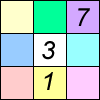Search by Topic

Resources tagged with Working systematically similar to Kangaroo Subtraction:

Filter by: Content type:
Age range:
Challenge level:

There are 127 results

Broad Topics > Using, Applying and Reasoning about Mathematics > Working systematicallyAge 7 to 14 Challenge Level:

I added together some of my neighbours' house numbers. Can you explain the patterns I noticed?How Old Are the Children?

Age 11 to 14 Challenge Level:

A student in a maths class was trying to get some information from her teacher. She was given some clues and then the teacher ended by saying, "Well, how old are they?"Football Sum

Age 11 to 14 Challenge Level:

Find the values of the nine letters in the sum: FOOT + BALL = GAMEAge 11 to 14 Challenge Level:

Replace the letters with numbers to make the addition work out correctly. R E A D + T H I S = P A G EMultiply the Addition Square

Age 11 to 14 Challenge Level:

If you take a three by three square on a 1-10 addition square and multiply the diagonally opposite numbers together, what is the difference between these products. Why?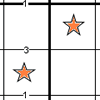Constellation Sudoku

Age 14 to 18 Challenge Level:

Special clue numbers related to the difference between numbers in two adjacent cells and values of the stars in the "constellation" make this a doubly interesting problem.Two and Two

Age 11 to 14 Challenge Level:

How many solutions can you find to this sum? Each of the different letters stands for a different number.9 Weights

Age 11 to 14 Challenge Level:

You have been given nine weights, one of which is slightly heavier than the rest. Can you work out which weight is heavier in just two weighings of the balance?Sticky Numbers

Age 11 to 14 Challenge Level:

Can you arrange the numbers 1 to 17 in a row so that each adjacent pair adds up to a square number?Cayley

Age 11 to 14 Challenge Level:

The letters in the following addition sum represent the digits 1 ... 9. If A=3 and D=2, what number is represented by "CAYLEY"?LCM Sudoku

Age 14 to 16 Challenge Level:

Here is a Sudoku with a difference! Use information about lowest common multiples to help you solve it.Cinema Problem

Age 11 to 14 Challenge Level:

A cinema has 100 seats. Show how it is possible to sell exactly 100 tickets and take exactly £100 if the prices are £10 for adults, 50p for pensioners and 10p for children.A First Product Sudoku

Age 11 to 14 Challenge Level:

Given the products of adjacent cells, can you complete this Sudoku?Window Frames

Age 5 to 14 Challenge Level:

This task encourages you to investigate the number of edging pieces and panes in different sized windows.Reach 100

Age 7 to 14 Challenge Level:

Choose four different digits from 1-9 and put one in each box so that the resulting four two-digit numbers add to a total of 100.Ben's Game

Age 11 to 14 Challenge Level:

Ben passed a third of his counters to Jack, Jack passed a quarter of his counters to Emma and Emma passed a fifth of her counters to Ben. After this they all had the same number of counters.Peaches Today, Peaches Tomorrow...

Age 11 to 14 Challenge Level:

Whenever a monkey has peaches, he always keeps a fraction of them each day, gives the rest away, and then eats one. How long could he make his peaches last for?Product Sudoku

Age 11 to 14 Challenge Level:

The clues for this Sudoku are the product of the numbers in adjacent squares.Consecutive Negative Numbers

Age 11 to 14 Challenge Level:

Do you notice anything about the solutions when you add and/or subtract consecutive negative numbers?Pole Star Sudoku 2

Age 11 to 16 Challenge Level:

This Sudoku, based on differences. Using the one clue number can you find the solution?American Billions

Age 11 to 14 Challenge Level:

Play the divisibility game to create numbers in which the first two digits make a number divisible by 2, the first three digits make a number divisible by 3...Special Numbers

Age 11 to 14 Challenge Level:

My two digit number is special because adding the sum of its digits to the product of its digits gives me my original number. What could my number be?Maths Trails

Age 7 to 14

The NRICH team are always looking for new ways to engage teachers and pupils in problem solving. Here we explain the thinking behind maths trails.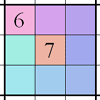Integrated Product Sudoku

Age 11 to 16 Challenge Level:

This Sudoku puzzle can be solved with the help of small clue-numbers on the border lines between pairs of neighbouring squares of the grid.Factor Lines

Age 7 to 14 Challenge Level:

Arrange the four number cards on the grid, according to the rules, to make a diagonal, vertical or horizontal line.LCM Sudoku II

Age 11 to 18 Challenge Level:

You are given the Lowest Common Multiples of sets of digits. Find the digits and then solve the Sudoku.More Magic Potting Sheds

Age 11 to 14 Challenge Level:

The number of plants in Mr McGregor's magic potting shed increases overnight. He'd like to put the same number of plants in each of his gardens, planting one garden each day. How can he do it?Star Product Sudoku

Age 11 to 16 Challenge Level:

The puzzle can be solved by finding the values of the unknown digits (all indicated by asterisks) in the squares of the $9\times9$ grid.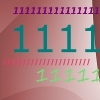Ones Only

Age 11 to 14 Challenge Level:

Find the smallest whole number which, when mutiplied by 7, gives a product consisting entirely of ones.Multiples Sudoku

Age 11 to 14 Challenge Level:

Each clue in this Sudoku is the product of the two numbers in adjacent cells.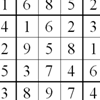Twin Chute-swapping Sudoku

Age 14 to 18 Challenge Level:

A pair of Sudokus with lots in common. In fact they are the same problem but rearranged. Can you find how they relate to solve them both?A Long Time at the Till

Age 14 to 18 Challenge Level:

Try to solve this very difficult problem and then study our two suggested solutions. How would you use your knowledge to try to solve variants on the original problem?Difference Dynamics

Age 14 to 18 Challenge Level:

Take three whole numbers. The differences between them give you three new numbers. Find the differences between the new numbers and keep repeating this. What happens?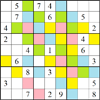Rainstorm Sudoku

Age 14 to 16 Challenge Level:

Use the clues about the shaded areas to help solve this sudokuSumming Consecutive Numbers

Age 11 to 14 Challenge Level:

15 = 7 + 8 and 10 = 1 + 2 + 3 + 4. Can you say which numbers can be expressed as the sum of two or more consecutive integers?Age 11 to 16 Challenge Level:

Four numbers on an intersection that need to be placed in the surrounding cells. That is all you need to know to solve this sudoku.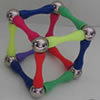Magnetic Personality

Age 7 to 16 Challenge Level:

60 pieces and a challenge. What can you make and how many of the pieces can you use creating skeleton polyhedra?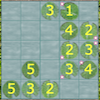Latin Lilies

Age 7 to 16 Challenge Level:

Have a go at this game which has been inspired by the Big Internet Math-Off 2019. Can you gain more columns of lily pads than your opponent?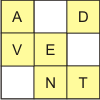Age 11 to 14 Challenge Level:

Rather than using the numbers 1-9, this sudoku uses the nine different letters used to make the words "Advent Calendar".Making Maths: Double-sided Magic Square

Age 7 to 14 Challenge Level:

Make your own double-sided magic square. But can you complete both sides once you've made the pieces?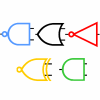Olympic Logic

Age 11 to 16 Challenge Level:

Can you use your powers of logic and deduction to work out the missing information in these sporty situations?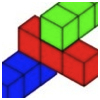Building with Longer Rods

Age 7 to 14 Challenge Level:

A challenging activity focusing on finding all possible ways of stacking rods.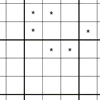Age 11 to 14 Challenge Level:

You need to find the values of the stars before you can apply normal Sudoku rules.More Plant Spaces

Age 7 to 14 Challenge Level:

This challenging activity involves finding different ways to distribute fifteen items among four sets, when the sets must include three, four, five and six items.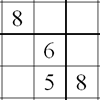Twin Line-swapping Sudoku

Age 14 to 16 Challenge Level:

A pair of Sudoku puzzles that together lead to a complete solution.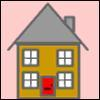Smith and Jones

Age 14 to 16 Challenge Level:

Mr Smith and Mr Jones are two maths teachers. By asking questions, the answers to which may be right or wrong, Mr Jones is able to find the number of the house Mr Smith lives in... Or not!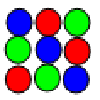Latin Squares

Age 11 to 18

A Latin square of order n is an array of n symbols in which each symbol occurs exactly once in each row and exactly once in each column.More Children and Plants

Age 7 to 14 Challenge Level:

This challenge extends the Plants investigation so now four or more children are involved.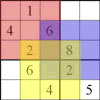Seasonal Twin Sudokus

Age 11 to 16 Challenge Level:

This pair of linked Sudokus matches letters with numbers and hides a seasonal greeting. Can you find it?